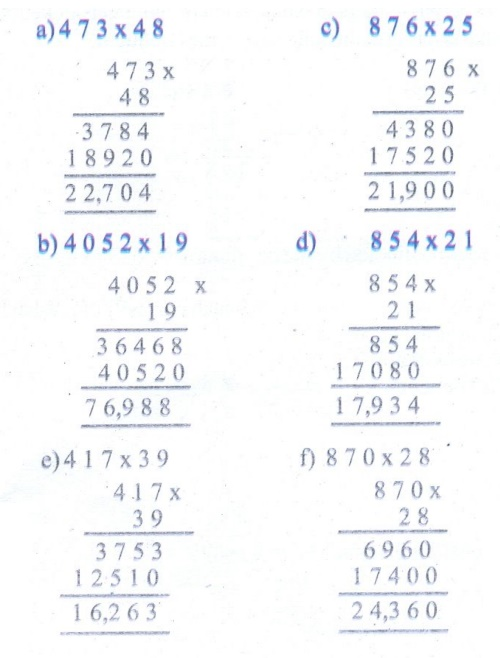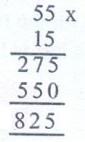Home | | Maths 5th Std | Exercise 2.7 (Multiplication)

# Exercise 2.7 (Multiplication)

Text Book Back Exercises Questions with Answers, Solution : 5th Maths : Term 1 Unit 2 : Numbers : Exercise 2.7 (Multiplication)

Exercise 2.7

1. Multiply:

a. 473 × 48

b. 4052 × 19

c. 876 × 25

d. 854 × 21

e. 417 × 39

f. 870 × 28a. There are 55 mangoes in a basket. Cost of one mango is ₹15. What is the total cost of 55 mangoes?

Cost of one mango = ₹ 15

The total cost of 55 mangoes = ₹ 55 × 15The total cost of 55 mangoes = ₹ 825

b. There are 55 passengers in a bus. Each of them gets tickets of ₹25. What is the amount collected by the conductor?

Fare of one ticket = ₹ 25

Fare of 55 tickets = ₹ 55 × 25Total amount collected by the conductor = ₹ 1375

c. A classroom has 23 benches. Each bench cost is ₹725. What is the total cast for 23 benches?

Cost of one bench = ₹ 725

Cost of 23 benches = ₹725 × 23The total cost for 23 benches = ₹ 16675

d. There arc 675 people living in a village. One person uses 25 L of water daily. How much water is need for the village every day?

One person needs water = 25 L

675 people need water = 675 × 25LWater needed for the village every day = 16,875 L

e. In a building, there are 26 rooms. The cost of painting for one room is ₹950. What is the total cost of painting the building?

Cost of painting for one room = ₹ 950

Cost of painting for 26 rooms = 950 × 26Total cost of painting the building = ₹ 24,700